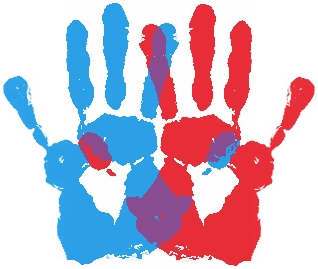Logic Level 1A class consists of 100 boys and 100 girls. A survey was conducted on everyone to determine whether there are more left-handed students or more right-handed students. Each student was either asked "Are you left-handed" or "Are you right-handned", and the results were tabulated as:

Boys: 24 out of 30 are left-handed, and 49 out of 70 are right-handed.

Girls: 28 out of 70 are left-handed, and 6 out of 30 are right-handed.

By tabulating the data, we have:

 Gender Left-handed Right-handed Boy $\frac{24}{30} = \mathbf{80\%}$ $\frac{49}{70} = 70\%$ Girl $\frac{28}{70} = \mathbf{40\%}$ $\frac{6}{30} = 20\%$

Is it true that there are more left-handed students than right-handed students in this class?

Note: Assume that no student is included in more than one statistical representation.

×